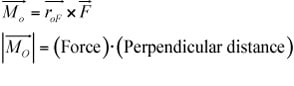##### Statics For Dummies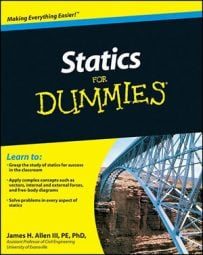As with any branch of physics, solving statics problems requires you to remember all sorts of calculations, diagrams, and formulas. The key to statics success, then, is keeping your shear and moment diagrams straight from your free-body diagrams and knowing the differences among the calculations for moments, centroids, vectors, and pressures.

## Checklist for solving statics problems

Solving statics problems can be complicated; each problem requires a list of items to account for and equations to create and solve. Solve statics problems with ease by using this checklist:

1. Draw a free-body diagram of the entire system.

In addition to dimensions and angles, you must include four major categories of items on a properly constructed free-body diagram:

Support reactions

Self weight

2. Write equilibrium equations to compute as many unknown support reactions as possible.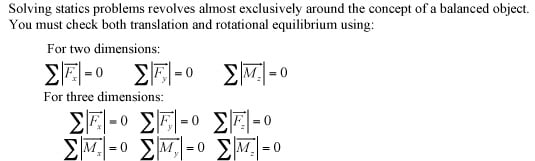3. To solve for internal forces, identify the type of structure and write your equilibrium equations.

After you identify the type of structure, you then know which technique to use to help you write your equilibrium equations:

Trusses/axial members: Members are loaded with internal axial forces only. To solve, you can use the method of joints or the method of sections.

Beams (bending members): Members are loaded with internal axial forces, shear forces, and moments. To solve, cut the member at the desired location, draw a new free-body diagram of the cut section, and write equilibrium equations.

Frames/machines: Members are loaded with internal axial forces, shear forces, and moments. To solve, use the blow-it-all-apart approach to break the structure into smaller pieces. Look for internal hinges as common places to separate your structure and draw free-body diagrams to solve for the connecting pin forces.

Cable structures: Members are constructed from axially loaded cables. Identify the type of cable loading (concentrated, parabolic/uniform, or catenary). Compute the cable tension at the location of known maximum sag (or vice versa).

Submerged surfaces: Members are subjected to fluid pressure. To solve, draw a free-body diagram of the hydrostatic pressure diagram which is zero at the fluid surface and linearly increases with depth. Include the weight of the fluid on objects with non-vertical faces.

## Computing submerged surface pressures

When working submerged surface problems in statics, remember that all submerged surfaces have a fluid acting upon them, causing pressure. You must compute two pressures: the hydrostatic pressure resultant and the fluid self weight.

• Hydrostatic pressure resultant: The hydrostatic pressure resultant acts horizontally at 0.333z (z is the depth of the fluid) from the bottom of the pressure distribution.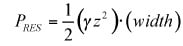• Fluid self weight: The fluid self weight is

acting downward at the centroid (geometric center) of the area of fluid.

## Calculating a region or load's center of area: Centroids

The centroid or center of area of a geometric region is the geometric center of an object’s shape. Centroid calculations are very common in statics, whether you’re calculating the location of a distributed load’s resultant or determining an object’s center of mass. To compute the center of area of a region (or distributed load), you can compute the x-coordinate (and the other coordinates similarly) from the following equations:

• For discrete regions: You can break discrete regions into simple shapes such as triangles, rectangles, circles, and so on.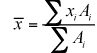For discrete shapes, creating a simple table such as the one that follows for each coordinate can be useful. You can create as many rows as you need for as many regions as you have.

### Sample Table for Calculating Centroids for Discrete Regions

 xi Ai xiAi Region 1 x-distance for Region 1 Area of Region 1 Product of xi and Ai Totals ——————- Sum of all Ai rows Sum of all xiAi rows

For continuous regions: Continuous regions are usually defined by more complex boundaries, so you must define them with mathematical equations such as the one that follows: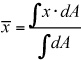## Developing shear and moment diagrams

Shear and moment diagrams are a statics tool that engineers create to determine the internal shear force and moments at all locations within an object. Start by locating the critical points and then sketching the shear diagram.

• Critical point locations:

• Start and stop of structure (extreme ends)

• Concentrated forces

• Concentrated moments

• Start and stop of distributed loads

• Internal hinges

• Support locations

• Points of zero shear (V = 0) — for moment diagrams only.

• Important features to remember when drawing the diagram:

• Concentrated forces cause an instant jump in shear.

• Concentrated moments cause an instant jump in moment.

• Order increases from load to shear to moment (that is, 1st order load diagram, 2nd order shear, 3rd order moment).

• The slope of the moment diagram is equal to the value of shear.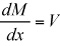• If the load area is positive, the change in shear is positive. If the shear area is positive, the change in moment is positive.

## Cartesian vector formulas for solving statics problems

In many statics problems, you must be able to quickly and efficiently create vectors in the Cartesian plane. Luckily, you can accomplish your Cartesian vector creations easily with the handy vector formulas in this list: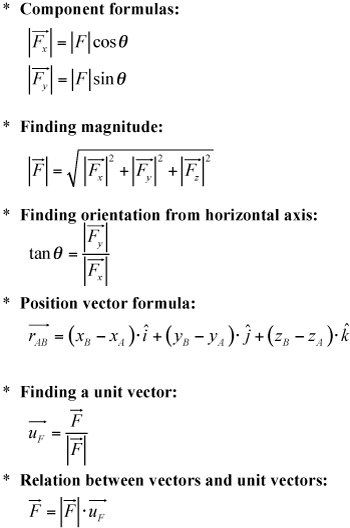Force vectors and distance vectors are the most basic vectors that you deal with.

## How to calculate a force's moment

In statics, moments are effects (of a force) that cause rotation. When computing equilibrium, you must be able to calculate a moment for every force on your free-body diagram. To determine a force’s moment, you use one of two different calculations, as you can see in the following list.

• Scalar calculation (for two dimensions): To calculate the moment about a Point O in scalar calculations, you need the magnitude of the force and the perpendicular distance from Point O to the line of action of the Force F.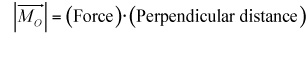• Vector calculation (for two or three dimensions): To compute the moment vector about a Point O in vector calculations, you must determine the Force F in Cartesian vector form and the position vector from Point O to the line of action of the Force F.